## Image Formation in Convex Mirrors

Image Formation in Convex Mirrors

While drawing images of the object we benefits from the special rays given above. We use them because we know the paths of them. In convex mirrors image is usually formed behind the mirror, it is virtual and erect. Location of the image is always between the focal point and vertex of the mirror. Look at the given pictures below, they show what I try to say.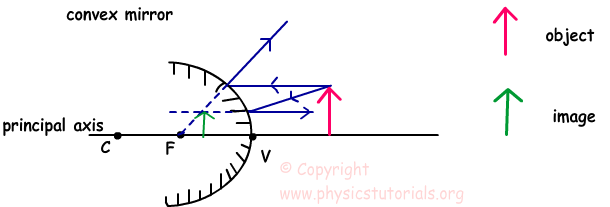We send two rays from the top of the object. One of the rays is parallel to the principal axis and reflects from the mirror as it comes from focal point and the extension of the second ray passes from the focal point so it travels parallel to the principal axis after reflection. Behind the mirror, extensions of the two reflected rays intersect at one point at which image of the object is located. As you can see from the picture, height of the image is smaller than the object, it is virtual and erect. Now look at another example given above.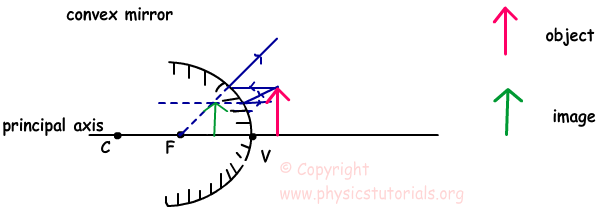In this picture, object is closer to the mirror. Image is again formed between the focal point and vertex. However, in this case height of the image is larger than the situation given above. We can conclude that, closer the object to the mirror bigger the height of the image. But, don’t forget, image is always smaller than the object.

The last example is given below in which object is at infinity and image is formed behind the mirror at focal point like a point.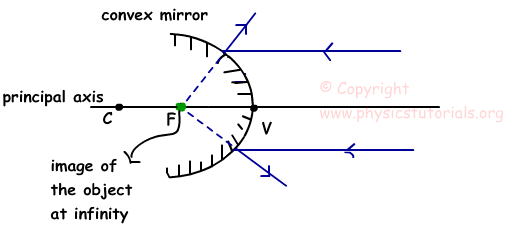Example: Plane mirror and convex mirror are placed on same principal axis. If the focal length of the convex mirror is 12 cm find the distance d shown in the picture.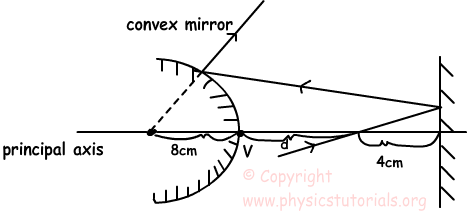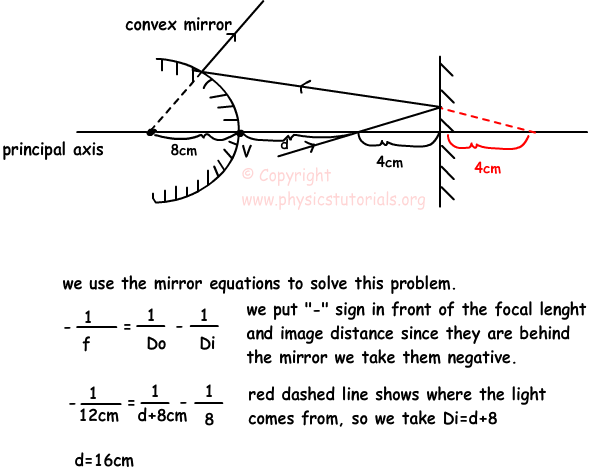Optics Exams and Solutions

Author:

Tags: Concave Mirrors |